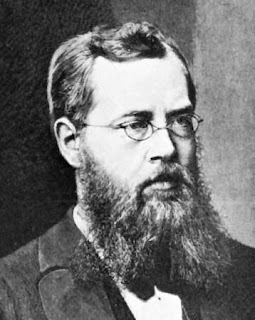## The Lie algebra of generators

Last time we derived the fundamental composition relationship which is the root cause of why complex numbers play a  key role in quantum mechanics. However the pattern:

Real          = real * real           -  imaginary * imaginary
Imaginary = imaginary * real + real * imaginary

can also be understood as:

Symmetric       = symmetric * symmetric       -  antisymmetric * antisymmetric
Antisymmetric = antisymmetric * symmetric + symmetric * antisymmetric

and when we look at it from this point of view we arrive at a Lie algebra.

Now let me start by giving a high level overview of Lie algebras. First, the name comes from Sophus Lie, a Norwegian mathematician (no, he was not Chinese :) ) who pursued to do in partial differential equation what Galois did for polynomial equations.Sophus Lie

Galois was using the permutation of solutions to determine if a particular polynomial equation is solvable. Instead of discrete symmetries, Lie was using continuous symmetries to simplify partial differential equations. From this came the idea of a continuous group (like the rotation group). In physics one encounters the gauge groups of the Standard Model as examples of Lie groups. In special relativity one encounters SO(1,3).

Linearizing the Lie group around the identity gives rise to what is called a Lie algebra. The elements of a Lie algebra are sometimes called generators. From the Lie algebra one may reconstruct its Lie group by exponentiation, but this does not always work as there can be distinct Lie groups with the same Lie algebra.

Now back on $$\alpha$$, is this a Lie algebra? It respects bilinearity and the Leibniz identity, but what about antisymmetry and Jacobi identity? It turns out that all we need is antisymmetry! We get the Jacobi identity for free because Leibniz + antisymmetry = Jacobi:

$$A \alpha (B\alpha C) = (A\alpha B) \alpha C + B\alpha (A \alpha C)$$

by antisymmetry:

$$A \alpha (B\alpha C) +C \alpha (A\alpha B) - B\alpha (A \alpha C)= 0$$
$$A \alpha (B\alpha C) +C \alpha (A\alpha B) + B\alpha (C \alpha A)= 0$$ q.e.d.

But how can we prove antisymmetry? By itself $$\alpha$$ is only a Loday algebra. However, invariance under composition comes to the rescue! So how can we do it? First , let's observe that in the Leibniz identity above the order of $$A$$ and $$B$$ terms occurs as $$AB$$ and as $$BA$$ and we want to take advantage of this. Second, by invariance under composition we can define the bipartite Leibniz identity:

$$(A_1 \otimes A_2) \alpha_{12} ((B_1 \otimes B_2) \alpha_{12} (C_1 \otimes C_2)) = ((A_1 \otimes A_2 ) \alpha_{12} (B_1 \otimes B_2)) \alpha_{12} (C_1 \otimes C_2)$$
$$+ (B_1 \otimes B_2 ) \alpha_{12} ((A_1 \otimes A_2) \alpha_{12} (C_1 \otimes C_2))$$

and now we want to use the fundamental composition relationship we derived last time:

$$\alpha_{12} = \alpha \otimes \sigma + \sigma \otimes \alpha$$

to expand the mess above. However, a great simplification occurs if we pick: $$B_1 = C_2 = 1$$ :

$$0 = (A_1 \alpha C_1) \otimes (A_2 \alpha B_2 + B_2 \alpha A_2)$$

which is valid for any $$A_1 \alpha C_1$$ Do it as an exercise to convince yourself it is true. Let me only show that the left hand side is zero:

$$((B_1 \otimes B_2) \alpha_{12} (C_1 \otimes C_2)) = B_1 \alpha C_1 \otimes B_2 \sigma C_2 + B_1 \sigma C_1 \otimes B_2 \alpha C_2$$

but because $$B_1 = 1$$: $$B_1 \alpha C_1 = 0$$ and because  $$C_2 = 1$$: $$B_2 \alpha C_2 = 0$$  and the LHS is zero.

Hence:

$$A\alpha B = - B\alpha A$$

So $$\alpha$$ is a Lie algebra. Moreover by the fundamental composition relationship $$\sigma$$ must be a symmetric product to preserve the antisymmetry of $$\alpha$$ under composition.

Now we are getting somewhere. We know that quantum mechanics is described by C* algebras which can be decomposed into Lie and Jordan algebras. We got the Lie part and we are almost there for the Jordan side. Please stay tuned.